Related Articles

# Designing finite automata for various operations like 1’s complement, 2’s complement

• Last Updated : 06 Aug, 2021

Transducers in Finite Automata(FA) means FA with Output.
There are two types of Machines for FA with Output.

1. Mealy Machine :
It is FA in which output is associated with each transition. (that means output depends on state and input).
2. Moore Machine :
It is FA in which output is associated with each state. (that means output depends on state only).

By using these machines, we can design finite automata for various operations like :-

• 1’s Complement.
• 2’s Complement.
• Increment by 1.
• Change the sign bit.
• Integer Divisibility Tester.
• Logical functions (XOR, OR, AND, NOT).
• Sum of present bit & previous bit etc.

1. 1’s Complement :

1’s Complement of a given binary number is obtained by simply inverting each bit.
i.e. 1 <–> 0 and 0 <–> 1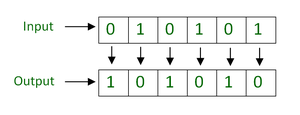Logic for Designing Using Mealy Machine –

• For input 0, output is 1.
• For input 1, output is 0.
So it requires only one state.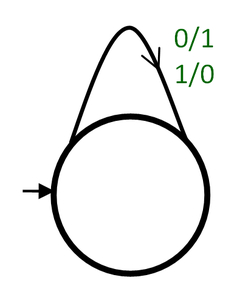1’s complement Mealy Machine

2. 2’s Complement :

Generally, 2’s complement is obtained by adding 1 to the 1’s complement of the number.
But for designing 2’s complement machine, we use another algorithm for deriving 2’s complement.

Algorithm –

• Start from LSB of the input.
• Copy the bit as it until the first ‘1’ comes.
• After that, complement each bit.

Example –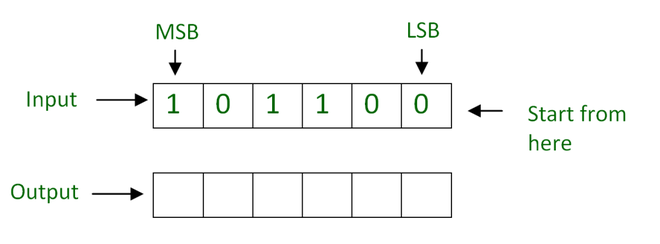Start from LSB of the input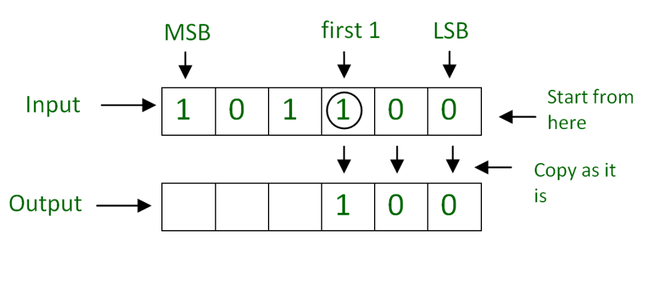Copy the bit as it until the first ‘1’ come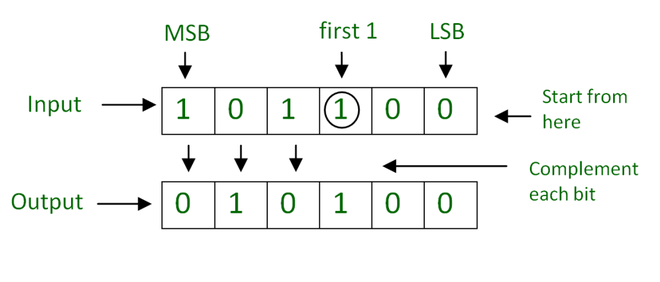Complement each bit

Logic for Designing Using Mealy Machine –

• Take input from LSB of the input.
• For every input till, the first 1 output is the same as the input.
• After that, for each input complement each bit using 1’s complement machine.

So, it requires 2 states. One state where output is the same as input and another state is 1’s complement state.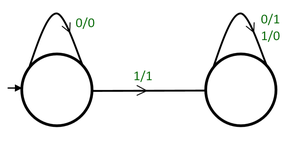2’s Complement Mealy Machine

When the first ‘1’ comes, it transfers to the next state to do complement of each bit.
Here, the behavior of the first state is to generate output the same as input and the behavior of the second state is to generate the complement.

The binary full adder adds 2 binary bits and carry input and generates sum output and carry output.

Working of Binary full adder –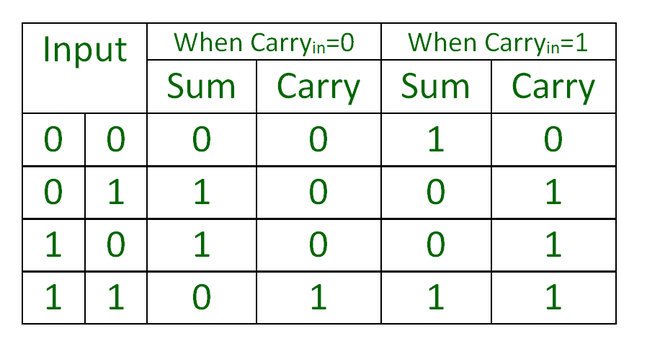This is a functionality table of the binary full adder. Depending on carry input, the output value of input is changes.

To design a Binary full adder, we use this table. According to this table there are two cases (carry=0 and carry=1) , so we need two states.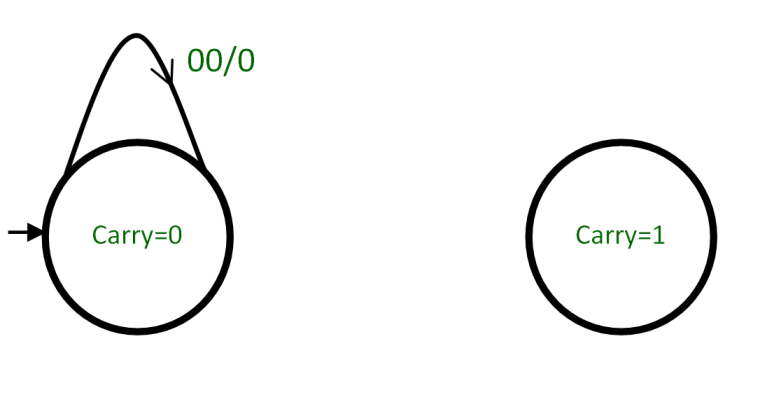When Carry=0 (From Carry=0 state ) On “00” input Sum=0  ( So, On 00 transition the output is 0 ) and Carry=0 ( So, it goes to Carry=0 state )

Design the rest of the machine according to the table. It looks like –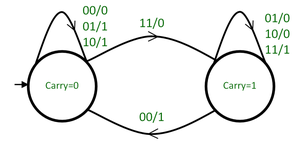4. Increment by 1 :
Increment by 1 means adding 1 to the binary number.
There are two cases in increment by 1 operation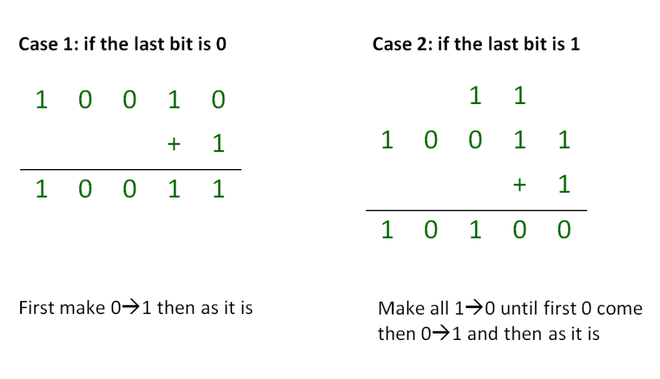Logic for Designing Machine –

On combining both cases, we got the algorithm that, until first 0 comes, makes all 10 and when 0 comes makes it 1, then the remaining part of the output is the same as the input. Here, inputs are taken from LSB.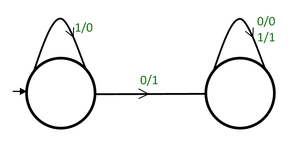Increment by 1 Mealy Machine

5. Change the sign bit :

Changing the sign bit is very simple. Just interchange the first bit and the other bits are the same as they are.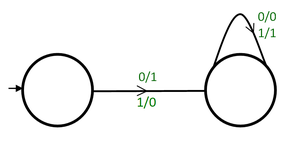Change the sign bit Mealy Machine

6. Integer Divisibility Tester :

The Integer Divisibility Tester Machine is the same as a Mod Machine, accepting the decimal value with output on the state.

Example –
3 Divisibility Tester Machine is the same as finite automata for  decimal value of string  mod 3=0 with output on the state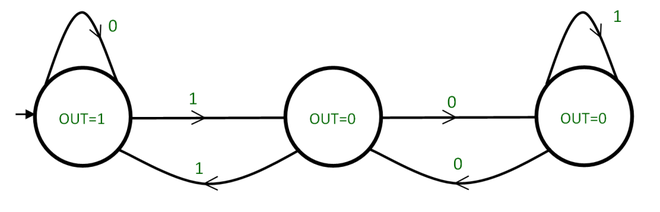3 Divisibility Tester Moore Machine

7. Logical functions (XOR, OR, AND, NOT) :

It is very easy to design machines for logic functions based on their logic tables.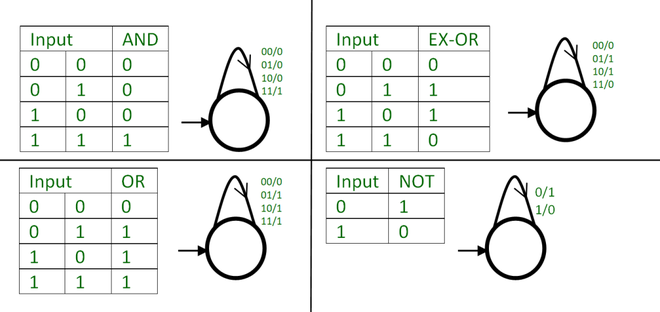Mealy Machines for different Logical Functions

8. Sum of present bit & previous bit :

If Previous bit= 0, On input 0, output = 00 and On input 1, output = 01
If Previous bit= 1, On input 0, output = 01 and On input 1, output = 10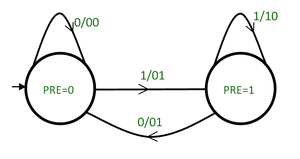Sum of present bit & previous bit

On pre=0, when there is a 1 transition, it moves to pre=1 because it has to the previous bit for the next bit. The same occurs in the case of  0 transition on pre=1

Attention reader! Don’t stop learning now.  Practice GATE exam well before the actual exam with the subject-wise and overall quizzes available in GATE Test Series Course.

Learn all GATE CS concepts with Free Live Classes on our youtube channel.

My Personal Notes arrow_drop_up